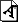# High-Performance Computing of Multiphase Flow

Multiphase flow problems are found in ocean and naval applications, chemical and other industrial and environmental processes, and in a variety of biomedical applications. In ocean engineering, wave-induced forces have a significant impact on structural and general hydrodynamic design. In particular, breaking waves may pose serious safety problems and affect the offshore structural integrity. Computational modelling of wave-induced hydrodynamics is often used in the design of conventional offshore structures in order to evaluate environmental loads. In the case of chemical applications, bubble formation or injection can be used to enhance mass transfer to improve chemical reactions. Bubble formation due to cavitation is also found in a range of pumping applications and may induce structural damage or loss of efficiency. Computational modelling is used to predict these types of multiphase flow phenomena.

The examples mentioned here are of the flow of two fluids separated by sharp interfaces. Numerically modelling advection of this interface is a challenging task and a range of methods have been developed over the last thirty years. The Volume of Fluid (VOF) method is a widely used approach that approximates the position, slope and curvature of the interface between two fluids using the volume fraction of each fluid. It is this volume fraction that is advected with the flowing fluid. The main difficulty of the VOF method is, to preserv the sharp interface during advection of fluid at the cell face.

Current research in our lab attempts to improve the models for this problem by modifying the VOF method. Although the VOF method is not necessarily CPU intensive, it imposes a further restriction on the solution of the fluid flow equations. The flow is almost exclusively unsteady and the time step generally needs to be significantly reduced by comparison with conventional fluid flow problems. This is particularly the case when surface tension must be taken into account. The sharp changes in the fluid properties at the interface also lead to algebraic systems that are more challenging to solve.

Mathematically the fluid properties and other phenomena are represented by a set of partial differential equations (PDEs) called the Navier-Stokes (NS) equations. In the process of numerical solution, PDEs are first converted to a large system of linear algebraic equations. The algebraic system of 'n' equations is represented by Ax = B, where 'A' is an n x n matrix and 'x' and 'B' are the n-dimensional vectors.

It is known that there exist two types of solvers  direct methods and iterative methods  for solving algebraic equations. Iterative methods are commonly employed to solve such large systems of equations, and are further classified into stationary and non-stationary types. In the non-stationary category, current variable values are updated based on several previous iteration values. The research being conducted at our lab employs the Krylov Subspace (KS) non-stationary methods. This work will investigate the power of some of KS methods such as the conjugate gradient method (CGM), bi-conjugate gradient method (bi-CGM), conjugate gradient stabilized method (CGSTAB) and bi-CGSTAB.

Solving these very large-scale systems is computationally very demanding and time-consuming, and requires a parallel or distributed environment such as a High-Peformance Computing Cluster (HPCC).

Due to the sharp change at the interface, matrices arising from PDEs are generally unsymmetric and ill-conditioned. It has been reported that the convergence of KS methods is slow when applied to those matrices. To overcome this difficulty, preconditioning techniques are applied to matrices in the solution procedure. In this project, preconditioned versions of KS methods have been developed in C++. Further, the parallel version of KS methods and preconditioners have also been developed using a message-passing library (MPI) and C++.Figure 1: Steps of the multiphase flow modelled on a parallel computer.

The objective of the project is to develop a numerical model based on the solution of the two- or three-dimensional NS equations to model two-fluid flow. The research focuses both on modelling moving boundaries and on improving the computational efficiency of the iterative methods used to solve the system of algebraic equations. The solution of the NS equations is coupled with the VOF method to simulate the motion of fluid. The parallel version of KS methods and preconditioners will be implemented on the parallel computer available at Dublin City University, and we propose a series of comparative stability, efficiency and scalability analyses of the parallel solver versus its serial version. The entire simulation process is illustrated in Figure 1.

E-mail: bkumarcomputing.dcu.ie